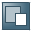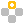Show TOC

###Data Collection Rules: CalculationsRules are used to calculate net mass, mass in supplementary unit, statistical values, and the total value of collected transactions.

##### Net Mass

Net mass is drawn from the Weight field of the document row. If the Weight field is blank, but you have defined the sales weight and the purchase weight for the item later, net mass is calculated as shown in the table below:

Transactional Type

Net Mass

Sales – A/R

Net Mass = Sales Weight * Quantity

Net Mass = Purchase Weight * QuantityNote

The sales weight and the purchase weight are drawn from the item master data. Rounding applies only to net mass, not to the sales weight or purchase weight of single items.

End of the note.
##### Mass in Supplementary Unit

Mass in supplementary unit is calculated for items to which you have assigned a supplementary unit.Note

If you want an item's mass in supplementary unit calculated, you must define a factor of supplementary unit in the item's Intrastat settings.

End of the note.

The weight value used in the calculation comes by default from the Weight field in the document. If the Weight field is blank, the weight value comes from the sales weight or the purchase weight in the item master data. Your setting of the Use Weight in Calculation of Mass in Supplementary Unit checkbox on the Intrastat Settings tab of the item master data also has an impact on the calculation.

The mass in supplementary unit is calculated in one of the following ways:

• If you use weight in the calculation, Mass in Supplementary Unit = Quantity × Factor of Supplementary Unit × Weight

• If you do not use weight in the calculation, Mass in Supplementary Unit = Quantity × Factor of Supplementary UnitExample

Calculation Examples of Mass in Supplementary Unit

Supplementary Unit

Quantity

Factor

Weight (kg)

Use Weight in Calculation

Formula/Result

Ton

5

0.001

10

Yes

Mass = 5 × 0.001 × 10 = 0.05

No

Mass = 5 × 0.001 = 0.005

0

Yes

Mass = 5 × 0.001 × 0 = 0

No

Mass = 5 × 0.001 = 0.005

End of the example.
##### Statistical Value

The statistical value of each transaction row depends on your setting of the Simplified Procedure checkbox on the General tab of the Intrastat Configuration window.

Calculation Rule for Statistical Values

Simplified Procedure checkbox

Calculation Rule

Not selected

Statistical Value = Row Total × Percentage Statistical Value of the Incoterms

Not selected and the document row is not assigned an Incoterms

Statistical Value = Row Total

Selected

##### Transaction Value and Total Declaration Value

The value of each declared transaction row is collected according to the following rules:

• By default, it is drawn from the Total (LC) field of each row, or the Total (Doc) field if the transaction is recorded in a foreign currency.

• If you have defined a discount for the document, the transaction value is the discounted row total.

• If a delivery or goods receipt PO is fully invoiced, the invoiced value is declared.

• If a partly invoiced delivery or goods receipt PO is included in a declaration, only its uninvoiced value is declared

The total declaration value is the sum of all collected transaction values. Depending on its value sign, a transaction value is deducted from or added to the total value.